# Practical exercise 2: THE MOON IN THE DISTANCE…HOW FAR??

In this task you will try to estimate the distance of the Moon from the Earth.

a) The gravitational acceleration6 at the Earth's surface is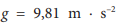and the Earth's radius R = 6 378 km. Determine the mass of the Earth from the Newton's law of universal gravitation.

b) The time of the Moon's orbit around the Earth is T = 27,3 days. Use the 3rd Kepler's law to estimate the mean distance of the Moon from the Earth (we neglect the mass of the Moon, which makes the calculation only approximate).Fig. 1 The Earth and the Moon in the same picture
(Source: https://www.nasa.gov/feature/goddard/from a million miles away nasa camera shows moon crossing face of earth , cit. 05.07.2018)

Solution:

a) The gravitational acceleration is determined from the Newton's law of universal gravitation. The gravitational force acting on an object of a mass m at the Earth's surface is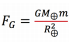.

The general relation for the force is given by the 2nd Newton's law F = mq, where q is the acceleration of an object7. If we denote the gravitational acceleration g, then from Newton's gravitational and second laws of motion we get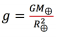From the relation we express the mass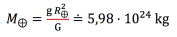The actual mass of the Earth is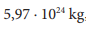, so our calculation is correct.

b) The orbital period is expressed in seconds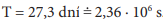In this task, we forget the mass of the Moon, which is not negligible in reality. We get an estimate of the mean distance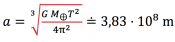.

The actual value of the semi major axis (middle distance) is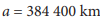Our calculation is almost accurate, which is due to the small mass of the Moon compared to the mass of the Earth,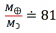applies.